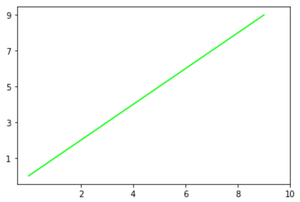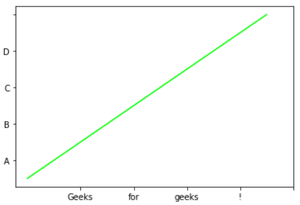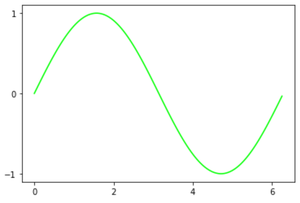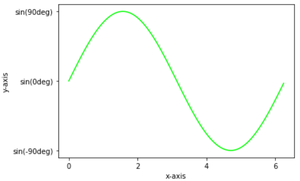Matplotlib – Setting Ticks and Tick Labels

• Last Updated : 26 Dec, 2020

In this article, we are going to discuss how set Ticks and Tick labels in a graph.

Ticks are the markers denoting data points on the axes and tick labels are the name given to ticks. By default matplotlib itself marks the data points on the axes but it has also provided us with setting their own axes having ticks and tick labels of their choice.

Methods used:

• plt.axes(*args, emit=True, **kwargs): For setting the axes for our plot with parameter rect as [left,bottom,width,height] for  setting axes position.
• plt.axes().set_xticks() and plt.axes().set_yticks() : For setting ticks on x-axis and y-axis respectively. having data in form of a list set as parameter.
• plt.axes().set_xlabels() and plt.axes().set_ylabels() : To set labels of our ticks with parameters in form of list.

Below are some examples which depict how to add ticks and ticklabels in a plot:

Example 1:

Python3

 # import required moduleimport matplotlib.pyplot as plt  # assign coordinatesx = y = [i for i in range(0, 10)]ax = plt.axes()  # depict illustrationplt.plot(x, y, color="lime")  # setting ticks for x-axisax.set_xticks([2, 4, 6, 8, 10])  # setting ticks for y-axisax.set_yticks([1, 3, 5, 7, 9])  plt.show()

Output:Example 2:

Python3

 # import required moduleimport matplotlib.pyplot as plt  # assign coordinatesx = y = [i for i in range(0, 10)]ax = plt.axes()  # depict illustrationplt.plot(x, y, color="lime")  # setting ticks for x-axisax.set_xticks([2, 4, 6, 8, 10])  # setting label for x tickax.set_xticklabels(['Geeks', 'for', 'geeks', '!'])  # setting ticks for y-axisax.set_yticks([1, 3, 5, 7, 9])  # setting label for y tickax.set_yticklabels(['A', 'B', 'C', 'D'])  plt.show()

Output:Example 3:

Python3

 # import required modulesimport matplotlib.pyplot as pltimport numpy as npimport math  # assign coordinatesx = np.arange(0, math.pi*2, 0.05)y = np.sin(x)ax = plt.axes()  # depict illustrationplt.plot(x, y, color="lime")  # setting ticks for x-axisax.set_xticks([0, 2, 4, 6])  # setting ticks for y-axisax.set_yticks([-1, 0, 1])  plt.show()

Output:Example 4:

Python3

 # import required modulesimport matplotlib.pyplot as pltimport numpy as npimport math  # assign coordinatesx = np.arange(0, math.pi*2, 0.05)y = np.sin(x)ax = plt.axes()plt.xlabel("x-axis")plt.ylabel("y-axis")  # depict illustrationplt.plot(x, y, color="lime")  # setting ticks for x-axisax.set_xticks([0, 2, 4, 6])  # setting ticks for y-axisax.set_yticks([-1, 0, 1])  # setting label for y tickax.set_yticklabels(["sin(-90deg)", "sin(0deg)", "sin(90deg)"])  plt.show()

Output:Attention geek! Strengthen your foundations with the Python Programming Foundation Course and learn the basics.

To begin with, your interview preparations Enhance your Data Structures concepts with the Python DS Course. And to begin with your Machine Learning Journey, join the Machine Learning - Basic Level Course

My Personal Notes arrow_drop_up